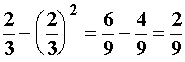LEGACY CONTENT. If you are looking for Voteview.com, PLEASE CLICK HERE

This site is an archived version of Voteview.com archived from University of Georgia on May 23, 2017. This point-in-time capture includes all files publicly linked on Voteview.com at that time. We provide access to this content as a service to ensure that past users of Voteview.com have access to historical files. This content will remain online until at least January 1st, 2018. UCLA provides no warranty or guarantee of access to these files.

45-733 Probability and Statistics I (3rd Mini AY 1999-2000 Flex-Mode and Flex-Time)

3.2 The sample space is:

S = {HH, HT, TH, TT}

and the corresponding values of Y are 2, -1, -1, and 1, respectively.

Now, since each outcome in S is equally likely

```                            { 1/2  y = -1
{
{ 1/4  y =  1
f(y) = {
{ 1/4  y =  2
{
{  0 otherwise
```

3.3 Since there are only 4 components, clearly Y = 2, 3, 4. Y = 2 occurs only if the first and second components tested are both defective. Let D indicate "Defective" and G indicate "Good". Hence:

P(Y = 2) = P(D Ç D) = (2/4)(1/3) = 1/6
P(Y = 3) = P(D Ç G Ç D) + P(G Ç D Ç D) = 2(2/4)(2/3)(1/2) = 2/6
P(Y = 4) = P(G Ç G Ç D Ç D) + P(D Ç G Ç G Ç D) + P(G Ç D Ç G Ç D) =
3(2/4)(1/3)(2/2) = 1/2

Hence, the distribution of Y is
```                            { 1/6  y = 2
{
{ 2/6  y = 3
f(y) = {
{ 3/6  y = 4
{
{  0 otherwise
```

3.5 Assume that the correct ordering of the animal words is ABC. If the child is guessing, there are 6 equally likely permutations she could choose, with Y = number of matches associated with each permutation". Therefore
```
A    B    C    Y
---------------------
A    B    C    3
A    C    B    1
B    A    C    1
B    C    A    0
C    A    B    0
C    B    A    1
```
Hence, P(0) = 2/6 = 1/3, P(1) = 3/6 = 1/2,
P(2) = 0, and P(3) = 1/6. The probability function is:
```                            { 1/3  y = 0
{
{ 1/2  y = 1
{
f(y) = {  0   y = 2
{
{ 1/6  y = 3
{
{  0 otherwise
```

3.17 Let X1 = # of contracts assigned to Firm 1; X2 = # of contracts assigned to Firm 2. There are 9 possible ways the contracts can be assigned to the 3 firms, each with a probability of 1/9. Considering the probability distributions for X1 and X2, we have,
```              { 4/9  x1 = 0              { 4/9  x2 = 0
{                          {
{ 4/9  x1 = 1              { 4/9  x2 = 1
f(x1) = {                  f(x2) = {
{ 1/9  x1 = 2              { 1/9  x2 = 2
{                          {
{  0 otherwise             {  0 otherwise
```

Hence, E(X1) = E(X2) = 0(4/9) + 1(4/9) + 2(1/9) = 6/9 = 2/3

Let Y = profit for Firm 1 = 90,000X1. Therefore, the expected profit is:

E[profit for Firm 1] = E(Y) = E[90,000X1] = 90,000E(X1) = 90,000(2/3) = 60,000

Now let W = profit for both Firm 1 and 2 = 90,000(X1 + X2). Thus the expected profit is:

E(W) = E[90,000(X1 + X2)] = 90,000E(X1 + X2) = 90,000[E(X1) + E(X2)] =
90,000[2/3 + 2/3] = 120,000

3.19 Let Y = "insurance company's loss in dollars". The probability distribution given is:
```                            { .001  y = 85,000
{
{ .01   y = 42,500
f(y) = {
{ .989  y = 0
{
{  0 otherwise
```

E(Loss) = E(Y) = .001*85,000 + .01*42,500 + .989*0 = 510

Hence, the insurance company should charge a premimum of \$510.00 for it to break exactly even.

3.22 Given

f(y) =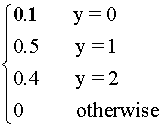Let X = Daily Cost = 10Y, then

E(Y) = 0*0.1 + 1*0.5 + 2*0.4 = 1.3
E(Y2) = 0*0.1 + 1*0.5 + 4*0.4 = 2.1
VAR(Y) = 2.1 - 1.32
Therefore: E(X)= E(10Y) = 10E(Y) = 10*(0*0.1 + 1*0.5 + 2*0.4) = 10*1.3 = \$13

and VAR(X) = VAR(10Y) = 100VAR(Y) = 100*.41 = 41

4.3 Given

f(y) =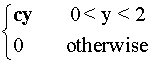1.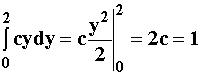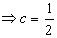2. F(y) = P(Y<y) =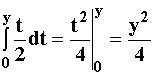Therefore:

F(y) =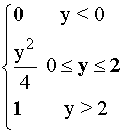3.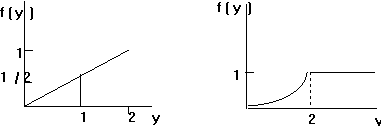4.        P[1 £ Y £ 2] = F(2) - F(1) = 1 -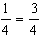5. Area of triangle =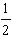* base * height = 1 -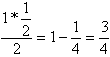4.6
1. Graph f(y)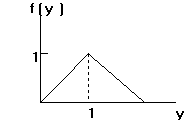2. For y < 0, F(y) = 0
For y > 2, F(y) = 2
For 0 < y < 1       F(y) = ò0ytdt = y2/2
For 1 £ y £ 2        F(y) = ò01tdt + ò1y(2 - t)dt = 1/2 + [2t - t2/2 |1y = 2y - y2/2 - 1
For y > 1 F(y) = 1

3. P[0.8£ Y£ 1.2] = F(1.2) - F(0.8) = (2.4 - 0.72 - 1) = 0.36

4. P(Y > 1.5 | Y > 1) = P(Y > 1.5)/P(Y > 1) = [1 - (3 - 1.125 -1)]/(1/2) = .25
4.8
1. Find c.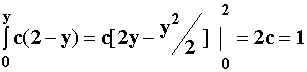Þ c =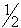2. F(y) = P(Y £ y) =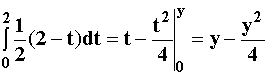Hence:

F(y) =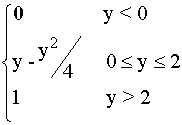3. Functions"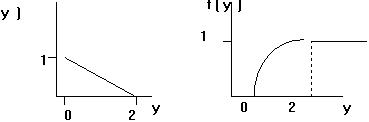4. P(1 £ Y £ 2) = F(2) - F(1) = 1 -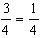5. Area of triangle =* base * height =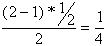4.12 f(y) =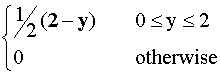E[Y] =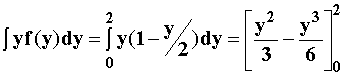=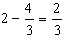E[Y2] =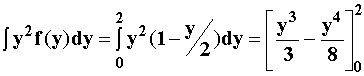=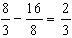VAR[Y] =